Hello Guest it is May 15, 2021, 02:11:32 PM

###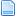Author Topic: Mach3 Sin() cos() tan() problem  (Read 933 times)

0 Members and 1 Guest are viewing this topic.

####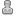rcaffin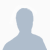•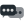1,035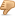##### Mach3 Sin() cos() tan() problem
« on: January 19, 2018, 05:15:42 AM »
Hi all

Just sometimes I want to machine around a curve which is not a circle, so I calculate the points using trigonometry. That is fine until I get to 360 degrees. In the real world
sin(360) = sin(0) = 0
tan(360) = tan(0) = 0
cos(90) = sin(0) = 0

On my Mach3 V0.062 I get something a little different.
sin(360+0.01) = 0.0001745  (correct)
sin(360) = -2.4492
cos(90 - 0.01) = 0.0001745  (correct)
cos(90) = 6.1230317

Of course, neither sin() nor cos() can ever be >1.000.

Has anyone else met this problem?

Cheers
Roger

####rcaffin•1,035##### Re: Mach3 Sin() cos() tan() problem
« Reply #1 on: January 20, 2018, 04:46:25 AM »
Some more details.
The problem seems to be with the G-code var monitor, NOT with the trig per se.
If I have the g-code var monitor active and type into the MDI line #1=123 I get 123 in the box for #1.
If I type #1=sin I get 0.5 in the box for #1.
If I type #1=sin I get -2.4492.
BUT
if I type g0 x[sin] the X DRO goes to 0! As it should.
Other tests match.

Which is an almighty PAIN, as a lot of my debugging involves (relies on) using the Var Monitor.

Cheers
Roger